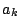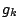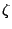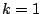# Explicit Formula

In the conjectures for mean-values there are two factors to notice: one is the arithmetical function, which arises in a quite natural way from the techniques of Dirichlet polynomials. The other is the factorwhich arises from Random Matrix theory.

These conjectures are a kind of pasting together of random matrix theory techniques and Dirichlet polynomial techniques.

An explanation for this phenomenon of disparate pieces is that the random matrix theory explains behavior of zeros clustered together in a spacing of approximately the inverse of the logarithm of the conductor, whereas it is quite likely that the arithmeticalarises from considerations of longer range correlations of the zeros of the family. The fact that thedo not appear from random matrix theory pose no problem from the point of view of the highest order main term of the asymptotic formula. However, we know that for the second and fourth moments ofthe main terms are actually polynomials of degrees 1 and 4, all terms of which can be expressed in closed form (See "Lower Order terms") . These terms seem to involve derivatives ofatand 2 as well as mysterious geometric factors that should be explainable from random matrix theory.

In order to really understand the mechanism connecting prime numbers, random matrices, and mean-value formulas, we will likely need to proceed via the explicit formula of Weil [ MR 14,727e] (see also Guinand [ MR 10,104g]). This formula is the explicit link between zero sums and prime number sums which will be needed to rigorously explain the connection between short and long range correlations.

Thus, we want a model of the Riemann zeta-function as a product of two factors: one of which is a kind of Euler product and the other is a kind of Hadamard product (whose behavior is like that of the characteristic polynomial of a random unitary matrix).

A related problem is to find a model for the distribution of zeros which works for both short and long range correlations. This would presumably include a random matrix model for the short range behavior, and some other model (somehow based on the prime numbers?) for the long range bahavior.

Back to the main index for L-functions and Random Matrix Theory.